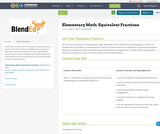Updating search results...

# 1 Result

View
Selected filters:
• NE.MA.4.1.1.I
RemixConditional Remix & Share Permitted
CC BY-NC-SA
Rating
0.0 stars

In this unit students will use models to show equivalent fractions. Students will use multiplication to generate equivalent fractions. Students will write and identify equivalent fractions in simplest form. Students will use equivalent fractions to represent a pair of fractions with a common denominator.

Subject:
Education
Material Type:
Activity/Lab
Assessment
Homework/Assignment
Lesson
Lesson Plan
Teaching/Learning Strategy
Unit of Study
Author:
Jessa Klute